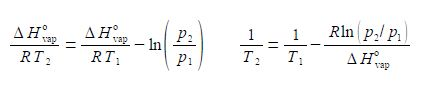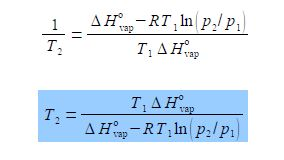# Theoretical Example of Vapour Pressure of Liquids

The gas phase pressure of a liquid is called its vapour pressure p and increases with increasing temperature of the liquidWhere ΔH0vap is the enthalpy of vaporization, the amount of heat required to vaporize a mole of liquid in its standard state; R is the gas constant; and T1 and T2 are low and high temperatures having p1 and p2 vapour pressures. Rearrange the equation to get T2 as the subject of the equation.

Solution

This question is not as easy as it first appears. The method suggested is one of several but with this type of question it is easy to make a mistake and obtain an incorrect solution, a step by step method is best.(1 )split the term in brackets into two terms; (2) cancel out top and bottom separately for each term inside the right hand bracket; (3) expand the bracket by multiplying it out;then (4) rearrange the equation so that the term containing T2 becomes the subject;

(5) multiply by R/ ΔH0vap throughout and

(6) then cancelling out top and bottom within each term;(7) before we can take the reciprocal on both sides we need to bring the right hand side to the common denominator T1 ΔH0vap, and finally

(8) we can now take reciprocals on both sides of the equation.